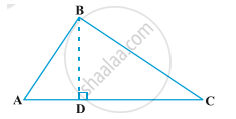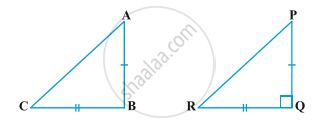Share

# Pythagoras Theorem

#### theorem

Theorem1: In a right triangle, the square of the hypotenuse is equal to the sum of the squares of the other two sides.Given: A right triangle ABC right angled at B.
Construction: Draw BD ⊥ AC
To prove: "AC"^2= "AB"^2+"BC"^2
∠A= ∠A (common)
∠BDA= ∠ABC= 90°

So, "BA"/"AC" = "AD"/"BA" (Corresponding parts of Congruent triangles)

"BA"^2 = "AC"xx "AD" .....eq1
Again, In ∆BCD and ∆BAC
∠C= ∠C (common)
and ∠BDC= ∠ABC= 90°
Therefore, ∆BCD ∼ ∆ACB (Angle-Angle similarity)

"BC"/"AC" = "CD"/"BC" (Corresponding parts of Congruent triangles)

"BC"^2= "AC"xx "CD" ......eq2
"BA"^2 + "BC"^2 = ("AC"xx "AD")+ ("AC"xx "CD")
= "AC" ("AD"+"CD")
= "AC" xx "AC"
"BA"^2 + "BC"^2 = "AC"^2
Hence proved.

Theorem2:  In a triangle, if square of one side is equal to the sum of the squares of the other two sides, then the angle opposite the first side is a right angle.Given: A triangle ABC such that "AC"^2= "AB"^2+"BC"^2
To prove: ∠B= 90°
Construction: Draw a triangle PQR such that PQ=AB, QR=BC and ∠Q=90°
Proof: In ∆PQR,
"PR"^2= "PQ"^2+"QR"^2 (Pythagoras theorem)
"PR"^2= "AB"^2 + "BC"^2 (by construction) .....eq1
"AC"^2= "AB"^2 + "BC"^2 (Given) .....eq2
From eq1 and eq2
Therefore, "PR"^2= "AC"^2
PR= AC
Now, In ∆ABC and ∆PQR,
AB= PQ (by construction)
BC= QR (by construction)
and AC= PR (proved above)
Therefore, ∆ABC ≅ ∆PQR (Side-Side-Side rule)
So, ∠B=∠Q= 90°  (Corresponding parts of Congruent triangles)
Therefore, ∠B= 90°
Hence proved.

#### description

• Pythagoras Theorem : In a right-angled triangle, the square on the hypotenuse is equal to the sum of the squares on the remaining two sides.
• In a right-angled triangle, the square on the hypotenuse is equal to the sum of the squares on the remaining two sides.

### Shaalaa.com

The Pythagorean Theorem and Its Converse [00:12:26]
S
2
0%

S